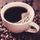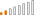cancel
Showing results for
Did you mean:Continued Contributor

## How to express Next Month First Day, Prev. Month First Day etc. (Time intelligence question)

Hi Community,

I am trying to write DAX on following examples (as shown below):Based on value of "Date" (like 02/20/2021, 01/10/2021), I would like to create column value like "Next Month First Day", "Current Month First Day", "2 Month..", "4 Month.." etc.

I think creating Column is what I needed now, but if there is a way to create Measure, I will use later.

Thanks.

1 ACCEPTED SOLUTIONSuper User

Hi:

If your matrix has month as column, then this would work as a measure:

Next Month First Day =
VAR dayone = CALCULATE(Max(Dates[Date]))
return

5 REPLIES 5Super User

Hi Justin:

Anoter variation you might like is:

=NEXTDAY(LASTDATE(Dates[Date]))

It's less code and works if you are set up by month. Just FYI. BillSuper User

Hi:

If your matrix has month as column, then this would work as a measure:

Next Month First Day =
VAR dayone = CALCULATE(Max(Dates[Date]))
returnContinued Contributor

Thank you so much! How do we express in Column by chance?Super User

Hi:

NP.

Begin Next Month =
VAR currdate = Dates[Date]
return
EOMONTH(currdate,0)+1

If it works well, please accept as solution. Thanks!Continued Contributor

I got this now in column:
Current Month First Day1 = EOMONTH(CALENDAR[Date] ,-1)+1

2 Month Ago First Day1 = EOMONTH(CALENDAR[Date] ,-3)+1